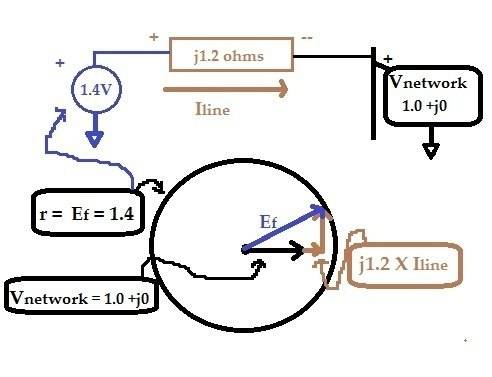# Power System, synchronous generator

LagCompensator
Sorry for bad title(little informative regarding my question), however I came across the following question given as an exam question at some university.

Question:
"A synchronous generator is running overexcited with excitation voltage Ef = 1.40 p.u and connected to the network at voltage of 1 p.u. This generator has synchronous reactance of 1.20 p.u., and delivering active power of 0.50 p.u. to the network. In the network, there is 1% increase in real power due to power drop from uncontrolled network connected renewable power source. Therefore, the prime mover (i.e. turbine) input is increased by 1%. The excitation or voltage controller of synchronous generator is not responding to this change. Explain how the reactive power delivery from the synchronous generator will change under this condition"

So, the Q delivered by the generator per phase is:
$Q = \frac{E_fV_t}{X_d}cos(\delta)-\frac{V_{t}^{2}}{X_d}-V_{t}^{2}(\frac{1}{X_d}-\frac{1}{X_q})sin^2(\delta)$ (1)
So according to this equation the Q will not change due to increase in turbine input.
However if we take a look at this equation:
$P = \frac{E_fV_t}{X_d}sin(\delta)-\frac{V_{t}^{2}}{X_d}-V_{t}^{2}(\frac{1}{X_d}-\frac{1}{X_q})sin(2\delta)$ (2)
An increase of P will cause an increase in $V_t$, and an increase of $V_t$ will increase the reactive power delivered by the sync. generator according to equation (1)

Can someone tell me if this is the right way of thinking or if I am missing something please tell me.

Best Regards

## Answers and Replies

Staff Emeritus
•cnh1995
Gold Member
Dearly Missed
later after i've checked my phasor arithmetic....

seems to me Vt is fixed by network at 1.0 and this is a (Vexc-Vnetwork)/jXs question.
You're given the real component before and after.

Gold Member
Dearly Missed
@LagCompensator
I'll take a stab at this.
Being not a power system guy it'll be amateurish
but i hope logical.
As textbooks and teaching methods evolve, some things become so refined that we lose track of the lower parts of the learning curve.
Question:
"A synchronous generator is running overexcited with excitation voltage Ef = 1.40 p.u and connected to the network at voltage of 1 p.u. This generator has synchronous reactance of 1.20 p.u., and delivering active power of 0.50 p.u. to the network. In the network, there is 1% increase in real power due to power drop from uncontrolled network connected renewable power source. Therefore, the prime mover (i.e. turbine) input is increased by 1%. The excitation or voltage controller of synchronous generator is not responding to this change. Explain how the reactive power delivery from the synchronous generator will change under this condition"

Okay , a picture might help us grunt this one out from the basics... I think in pictures not formulas.
Here's how i envision that circuit.
Circle at bottom is phasor diagram,
since we don't know power angle of the machine (angle of Ef) i just drew Ef some arbitrary place on a circle with radius 1.4.What do we know ?
Vnetwork i assumed infinite bus since they gave us no network impedance value.
I gave it value 1∠0 so it'll be our reference for phasor diagram. Polar for 1∠0 is 1+j0 .

We know that Iline has real component 0.5 because power is 0.5 .
So it'll have imaginary component jVars.
I'll assume lagging current, so that'll be -jVars. If i'm wrong we'll just get a negative Var current. Iline = 0.5 -jVars

Ohm's law tells us voltage across Zline is Iline X Zline.

Kirchoff's voltage law tells us that
Vnetwork + Iline X Zline = Vf
but we have a lot of unknown angles.......

Let's plug in what we know
Vnetwork + Iline X Zline = Vf
1+j0 + (0.5 -jVars) X (j1.2) = 1.4∠unknown
1 +j0.6 +1.2XVars = 1.4∠unknown
Aha !
Look back at the phasor diagram
we have a right triangle with adjacent side (1.0 + 1.2XVars) volts, opposite side 0.6 volts, hypotenuse 1.4 volts and no angles known.
Pythagoras lets us find adjacent side
(1.0 +1.2XVars) = √(1.42 - 0.62)
1.2 X Vars = 1.265 -1
so Vars = (1.265 - 1) / 1.2 = 0.2207

Repeating for real component of Iline = 0.51 i get 0.2159

That's the simplistic approach i'd use. If we worked numerous problems a shortcut would probably show its head..

Maybe some kind soul will improve(or correct) my explanation...

Last edited:
•cnh1995
LagCompensator
Thank you, i'll take a closer look once I get home from work.